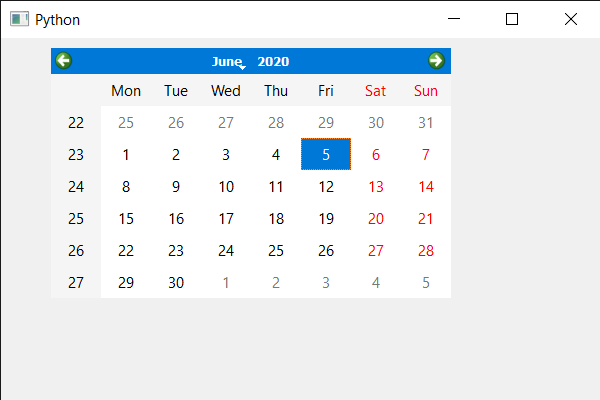Related Articles
PyQt5 QCalendarWidget – Assigning Base Size value
• Last Updated : 08 Jun, 2020

In this article we will see how we can set the base size value to the QCalendarWidget. By deafult base size has value zero for both width and height, base size is used to calculate a proper calendar size if the calendar defines sizeIncrement i.e its size change when window size changes. Base size is the initial size of the calnedar.

In order to do this we will use `setBaseSize` method with the QCalendarWidget object.

Syntax : calendar.setBaseSize(width, height)

Argument : It takes two integer as argument

Return : It return None

Below is the implementation

 `# importing libraries``from` `PyQt5.QtWidgets ``import` `*` `from` `PyQt5 ``import` `QtCore, QtGui``from` `PyQt5.QtGui ``import` `*` `from` `PyQt5.QtCore ``import` `*` `import` `sys`` ` ` ` `class` `Window(QMainWindow):`` ` `    ``def` `__init__(``self``):``        ``super``().__init__()`` ` `        ``# setting title``        ``self``.setWindowTitle(``"Python "``)`` ` `        ``# setting geometry``        ``self``.setGeometry(``100``, ``100``, ``600``, ``400``)`` ` `        ``# calling method``        ``self``.UiComponents()`` ` `        ``# showing all the widgets``        ``self``.show()`` ` `    ``# method for components``    ``def` `UiComponents(``self``):`` ` `        ``# creating a QCalendarWidget object``        ``self``.calender ``=` `QCalendarWidget(``self``)`` ` `        ``# setting geometry to the calender``        ``self``.calender.setGeometry(``50``, ``10``, ``400``, ``250``)`` ` `        ``# setting base size``        ``self``.calender.setBaseSize(``400``, ``25``)`` ` ` ` ` ` `# create pyqt5 app``App ``=` `QApplication(sys.argv)`` ` `# create the instance of our Window``window ``=` `Window()`` ` ` ` `# start the app``sys.exit(App.``exec``())`

Output :Attention geek! Strengthen your foundations with the Python Programming Foundation Course and learn the basics.

To begin with, your interview preparations Enhance your Data Structures concepts with the Python DS Course. And to begin with your Machine Learning Journey, join the Machine Learning – Basic Level Course

My Personal Notes arrow_drop_up